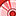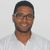# QlikView App Dev

Discussion Board for collaboration related to QlikView App Development.

cancel
Showing results for
Did you mean:Contributor

## Help with using crosstables in resident load

Hi,

I have 3 fields: Item, Type and Value. Each item has 2 types, A and B. I would like to have 3 fields: Item, A and B so A would be Value when type is A and B would be Value when type is B. In other words I would like to get rid of Type field so I could sum Type A and B values separately easily. How would I do this, with crosstable?

1 Solution

Accepted SolutionsSpecialist II

Hi,

I think crosstable would be going the other way, so taking 2 fields A & B & turning them into a type fields, this sounds more like a pivot, so something like;

``````Load
Dim,
Sum(If(Type='A',Value)) AS A,
Sum(If(Type='B',Value)) AS B
Group by Dim;
Dim, Type, Value
X, A, 10
X, B, 5
Y, A, 20
Y, B, 15
];``````

Cheers,

Chris.

2 RepliesSpecialist II

Hi,

I think crosstable would be going the other way, so taking 2 fields A & B & turning them into a type fields, this sounds more like a pivot, so something like;

``````Load
Dim,
Sum(If(Type='A',Value)) AS A,
Sum(If(Type='B',Value)) AS B
Group by Dim;
Dim, Type, Value
X, A, 10
X, B, 5
Y, A, 20
Y, B, 15
];``````

Cheers,

Chris.MVP

@pgkrsk   by using Generic Load, like :

``````Input:
Item, Type, Value
1, A, 10
2, B, 11
3, A, 12
4, B, 13
];

DATA:
Item,
Type,
Value

Resident Input;

CombinedGenericTable:

Drop Table   Input;

FOR i = NoOfTables()-1 to 0 STEP -1

LET vTable=TableName(\$(i));
//trace \$(i) - '\$(vTable)';
IF WildMatch('\$(vTable)', 'DATA.*') THEN

LEFT JOIN ([CombinedGenericTable]) LOAD distinct * RESIDENT [\$(vTable)];

DROP TABLE [\$(vTable)];

ENDIF

NEXT i``````

output:Regards,
Taoufiq ZARRA

"Please LIKE posts and "Accept as Solution" if the provided solution is helpful "

(you can mark up to 3 "solutions") 😉